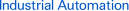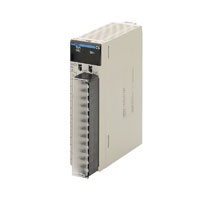# CS1W-PPS01

SYSMAC CS-series Process Analog I/O Units## Provides the functionality of isolators, power supplies, signal converters, and other devices.

#### General Specifications

The specifications shown in the following table apply to all the CS-series Process Analog I/O Units.

 Item Specification CS-series PLCs CS-series Special I/O Unit Backplane-mounted, single slot size 35 × 130 × 126 mm (W × H × D) 450 g max. 21-point removable terminal block (M3 screws, Tightening torque: 0.5 Nm) 00 to 95 Results of self-diagnosis shown on indicators. CPU Rack or CS-series Expansion Rack 80 Units (10 Units × 8 Racks)Confirm that the total current consumption of all the Units (including the CPU Unit) mountedto a single CPU Rack or Expansion Rack does not exceed the maximum power supply capacityof the Power Supply Unit. 0 to 55 ° C 10% to 90% (with no condensation)

#### Current consumption

 Name Current consumption (power) 5 V Model 26 V Isolated-type Pulse Input Unit CS1W-PPS01 0.20 A (1.00 W) 0.16 A (4.2 W)

#### (Reference) Maximum current and total power supplied

 Power Supply Unit Maximum total power 5 V 24 V Maximum current supplied (power) 26 V C200HW-PA204 4.6 A (23 W) 0.6 A (15.6 W) None 30 W C200HW-PA204C 4.6 A (23 W) 0.6 A (15.6 W) None 30 W C200HW-PA204S 4.6 A (23 W) 0.6 A (15.6 W) 0.8 A (19.2 W) 30 W C200HW-PA204R 4.6 A (23 W) 0.6 A (15.6 W) None 30 W C200HW-PD024 4.6 A (23 W) 0.6 A (15.6 W) None 30 W C200HW-PA209R 9 A (45 W) 1.3 A (33.8 W) None 45 W C200HW-PD025 5.3 A 1.3 A None 40 W CS1D-PA207R 7 A (35 W) 1.3 A (33.8 W) None 35 W CS1D-PD024 4.3 A (21.5 W) 0.56 A (14.6 W) None 28 W CS1D-PD025 5.3 A 1.3 A None 40 W

#### CS1W-PPS01 Isolated-type Pulse Input Unit

 Item Model Applicable PLC Specifications CS1W-PPS01 CS-series CS-series Special I/O Unit CS-series CPU Rack or CS-series Expansion Rack (Cannot be mounted to C200H Expansion I/O Rack orSYSMAC BUS Remote I/O Slave Rack.) 80 (within the allowable current consumption and power consumption range) 00 to 95 (Cannot duplicate Special I/O Unit numbers.) Special I/OUnit Area 10 words/UnitPulse Input Unit to CPU Unit:All process values, process value alarms (LL, L, H, HH), accumulated values, Accumulation Reset Bit 100 words/UnitCPU Unit to Pulse Input Unit:Instantaneous value conversion coefficient, instantaneous value scaling, pulse weight, number of valuesfor moving average, instantaneous value alarm settings (LL, L, H, HH), zero/span adjustment, etc. 4 Voltage input, no-voltage semiconductor input, contact input (selected individually for each of 4 inputs,according to connection terminals) No-voltage semiconductor input: Connected to voltage input terminals (between Fn+ and COMn).Maximum coefficient speed: 20,000 pulses/s (duty ratio: 50%)Detection voltage: 4 V DCShort-circuit current between terminals: 1.2 mA DCON resistance: 0.8 k Ω max.OFF resistance: 5.0 k Ω min. Voltage input: Connected to voltage input terminals (between Fn+ and COMn).Waveform: Square waveMaximum coefficient speed: 20,000 pulses/s (duty ratio: 50%)ON voltage: 0 to 1 VOFF voltage: 3 to 30 V Contact input: Connected to contact input terminals (between Sn+ and COMn).Maximum coefficient speed: 20 pulses/s (duty ratio: 50%)Detection voltage: 8 V DCShort-circuit current between terminals: 2.4 mA DCON resistance: 0.8 k Ω max.OFF resistance: 5.0 k Ω min. For no-voltage semiconductor inputs, etc., a 12-V DC power supply can be provided for the sensorsthat are the pulse sources.Output voltage: 12 V DC ± 15%Current capacity: 30 mA max.Limit current when short-circuited: 31 to 55 mAAllowable short-circuit time: No limit 100 ms/4 inputs Conversion period + one CPU Unit cycle Instantaneousvalue output Conversiontoinstantaneousvalues This function can be used to count the number of pulses per time unit, and to convertthe values to instantaneous values (pulses x pulse weight / time unit). Any of thefollowing can be selected as the time unit: 1 s, 3 s, 10 s, 30 s, or 60 s. (The time unit isset in the DM Area.)Note 1. Errors and fluctuations will increase when the input signal pulse rate is low, sospecify a longer time unit.Note 2. The instantaneous value is only refreshed at intervals of the time unit set.Therefore, when the Unit is restarted, the instantaneous value will be 0000 until the timeset as the time unit has elapsed.Note 3. When pulse weight conversion is used for accumulated values, the number ofpulses obtained by multiplying the actual number of input pulses by the pulse weight(0.1000 to 3.2000) for one time unit is used. Instantaneousvalue scaling This function can be used for scaling instantaneousvalues (pulses × pulse weight/time unit), i.e., settingdata with respect to a maximum value, and storingthem in the allocated words of the CIO Area.When instantaneous value (pulses × pulse weight/time unit) is 100% input: Can be set from 0.001pulses/time unit to 32,000 pulses/time unit.Maximum value for Instantaneous value scaling(industrial units): Scaling of the above instantaneousvalue (100% input) is possible from - 32,000 to32,000 (8300 to FFFF, 0000 to 7D00 hex).Note: When pulse weight conversion is used foraccumulated values, scaling is already performed foreach pulse, so an exponent of 10 of the industrialunit is set in the instantaneous value (pulses ×pulse weight per time unit) for a 100% input. Example 1:To obtain a pulse input of 0 to2,000 pulses/s for a flow of 0 to300.0 ml/s: Time unit: 1 sInstantaneous value 100% input:2,000Maximum value for instantaneousvalue scaling (industrial units):3,000Example 2:When pulse inputs at 0 to 2,000pulses/s are obtained for aflowrate of 0 to 300.0 ml/s, andthe pulse weight function is usedfor totaling: There are 0.15 ml perpulse, so the pulse weight = 0.15.For a flowrate of 0 to 300.0 ml/s,0 to 2,000 × 0.15 = 300 pulses/s.Therefore, Time unit: 1 sInstantaneous value 100% input:300Maximum value for instantaneousvalue scaling (industrial units):3,000 Data storagein the CIOArea The value derived from carrying out the followingprocessing in order of the instantaneous value(pulses x pulse weight/time unit) is stored in fourdigits hexadecimal (binary values) in the allocatedwords in the CIO Area.1) Mean value processing → 2) Instantaneousvalue scaling → 3) Scaling → 4) Zero/spanadjustment → 5) Output limits Mean valueprocessing(input filter) Calculates the moving average for the specified number of past instantaneous values(1 to 16), and stores that value in the CIO Area as the instantaneous value. Instantaneousvalue alarm Instantaneous value 4-point alarm (HH, H, L, LL), hysteresis, and ON-delay timer (0 to60 s) are available. Pulse weightconversion Performs scaling for a single pulse.Use for the accumulated value when the pulse weight (weight/pulse) is a fraction (not anexponent of 10). (See note.)The pulse weight (0.1 to 3.2) is multiplied by the actual number of pulses input. Thisnumber of pulses is used as the input for conversion to instantaneous values (pulses ×pulse weight per time unit) and the input for totaling prior to stepdown.Example: When the pulse weight from the flowmeter is 0.26 ml/pulse, the pulse weight isset to 0.26. When one pulse (0.26 ml) is input, it is treated as a 0.26 pulse, and when twopulses (0.52 ml) are input, they are treated as a 0.52 pulse.The weight per pulse becomes 1 ml, so to calculate in the CPU Unit the simple (unscaled)value in industrial units (ml) based on the accumulated value from the Pulse Input Unit(value in words n+5 to n+8), the value can be calculated simply using 1 ml/pulse.Note: When the accumulated value from the Pulse Input Unit in the CPU Unit is not used(i.e., when only the instantaneous value is used), pulse weight conversion is not required.Use instantaneousness value scaling to convert to industrial units. Accumulatedvalue The accumulated number of pulses (0 to 9,999 pulses) for each input is stored in theallocated words of the CIO Area. When 9,999 is exceeded, the value returns to 0 andstarts counting again.Note: When pulse weight conversion is used, the accumulated value for the number ofpulses obtained by multiplying the actual number of input pulses by the pulse weight(0.1000 to 3.2000) is used. Stepdown When the accumulated value is used, this function prevents accumulated value overflowby reducing the number of input pulses. The actual number of input pulses is multiplied byone of four factors ( × 1, × 0.1, × 0.01, or × 0.001), and the number of input pulsesaccumulated is then based on that value.Note: This stepdown function operates only for accumulated values, and not forinstantaneous values. When the pulse weight conversion function is used, it uses for thenumber of pulses obtained by multiplying the actual number of input pulses by the pulseweight (0.1000 to 3.2000). Between inputs and between input terminals and PLC signals: Isolation by transformer and photocoupler 20 M Ω (at 500 V DC) between inputs Between inputs: 1,000 V AC, at 50/60 Hz, for 1 min, leakage current 10 mA max. Terminal block (detachable) Set by rotary switches on front panel, from 0 to 95. Three LED indicators on front panel (for normal operation, errors detected at the Pulse Input Unit, anderrors related to the CPU Unit). Sensor input connector terminal block (detachable) 0.3 ms 5 V DC at 200 mA max., 26 V DC at 160 mA max. 10 min 35 × 130 × 126 mm (W × H × D)Note:The height including the Backplane is 145 mm. 450 g max. None